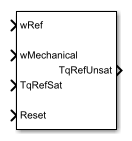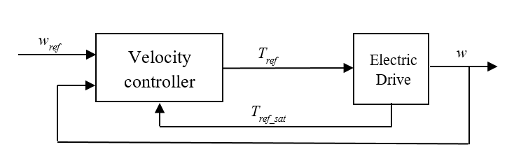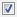# Velocity Controller

Discrete-time velocity controller

•Libraries:
Simscape / Electrical / Control / General Machine Control

## Description

The Velocity Controller block implements a velocity controller in discrete-time.

You provide measured and reference rotor velocities (w and wref) as inputs to the block. The block then outputs a reference torque Tref for an electric drive.To prevent windup in the integrator, feed the saturated reference torque Tref_sat from the electric drive back to the velocity controller.

### Equations

You can control the rotor angular velocity with discrete sample time Ts using one of three common approaches:

• Proportional-integral (PI) control, with proportional and integral gains Kp_w and Ki_w:

`${T}_{ref}=\left({K}_{p_w}+{K}_{i_w}\frac{{T}_{s}z}{z-1}\right)\left({w}_{ref}-w\right)$`

• Proportional (P) control, with proportional gain Kp_w:

`${T}_{ref}={K}_{p_w}\left({w}_{ref}-w\right)$`

• P-PI control characterized by a double velocity feedback loop as shown in the following figure:Here, the PI Controller block is structured as in the PI control strategy, and Kv is the proportional gain for a P controller.

Zero Cancellation

Using PI control results in a zero in the closed-loop transfer function, which can result in undesired overshoot in the closed-loop response. This zero can be canceled by introducing a zero-cancelation block in the feedforward path. The zero cancellation transfer function in discrete time is

`${G}_{ZC_w}\left(z\right)=\frac{\frac{{T}_{s}{K}_{i_w}}{{K}_{p_w}}}{z+\left(\frac{{T}_{s}-\frac{{K}_{p_w}}{{K}_{i_w}}}{\frac{{K}_{p_w}}{{K}_{i_w}}}\right)}$`

## Ports

### Input

expand all

Desired or reference velocity, in rad/s.

Data Types: `single` | `double`

Data Types: `single` | `double`

Saturated torque reference used for integral anti-windup gain, in N*m.

Data Types: `single` | `double`

External reset signal (rising edge) for the integrator.

Data Types: `single` | `double`

### Output

expand all

Unsaturated reference torque, in N*m.

Data Types: `single` | `double`

## Parameters

expand all

Type of controller:

• `PI control` — Proportional-integral control using a single feedback loop

• `P control` — Proportional-integral control using a single feedback loop

• `P-PI control` — Proportional and proportional-integral control using a double feedback loop

#### Dependencies

The Control type options affect the visibility or configurability of these parameters:

• Controller integral gain

• P controller proportional gain

• Anti-windup gain

• Integral anti-windup gain

• Sample time (-1 for inherited)

• Enable zero cancellation

Proportional gain for the:

• PI controller

• P controller in the single-loop control model

• PI controller in the P-PI controller

Integral gain for the PI or P-PI controller.

#### Dependencies

This parameter is visible only when the Control type is set to `PI control` or ```P-PI control```.

Proportional gain for the P controller in the P-PI controller.

#### Dependencies

This parameter is visible only when the is set to `P-PI control`.

Anti-windup gain for the PI controller.

#### Dependencies

This parameter is visible only when the is set to `PI control` or ```P-PI control```.

Time, in s, between consecutive block executions. During execution, the block produces outputs and, if appropriate, updates its internal state. For more information, see What Is Sample Time? and Specify Sample Time.

If this block is inside a triggered subsystem, inherit the sample time by setting this parameter to `-1`. If this block is in a continuous variable-step model, specify the sample time explicitly using a positive scalar.

#### Dependencies

This parameter is visible only when the is set to `PI control` or ```P-PI control```.

Time, in s, between consecutive discretizations. Discretization is required for zero cancellation.

#### Dependencies

This parameter is only visible when all these conditions are met:

• is set to ```PI control``` or ```P-PI control```.

• is set to `-1`.

• Enable zero cancellation is selected.

Option to use zero cancellation on the feedforward path.

#### Dependencies

The Enable zero cancellation parameter is visible only when is set to ```PI control``` or `P-PI control`.

The Discretization sample time parameter is only visible when Enable zero cancellation is selected.

 Naouar, M. W., A. A. Naassani, E. Monmasson, and I. Slama-Belkhodja. "FPGA-based predictive current controller for synchronous machine speed drive." IEEE Transactions on Power Electronics. Vol. 23, Number 4, 2008, pp. 2115–2126.